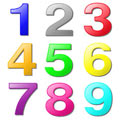The smallest number that can be divided exactly by 2, 3 and 4 is 12.

What is the smallest number that can be divided exactly by the numbers 2, 3, 4, 5 and 6?

What is the smallest number that can be divided exactly by the numbers 2, 3, 4, 5, 6, 7, 8 and 9?

Tagged with: